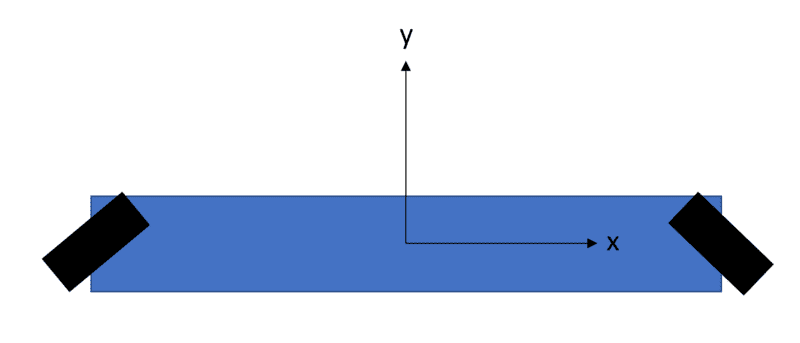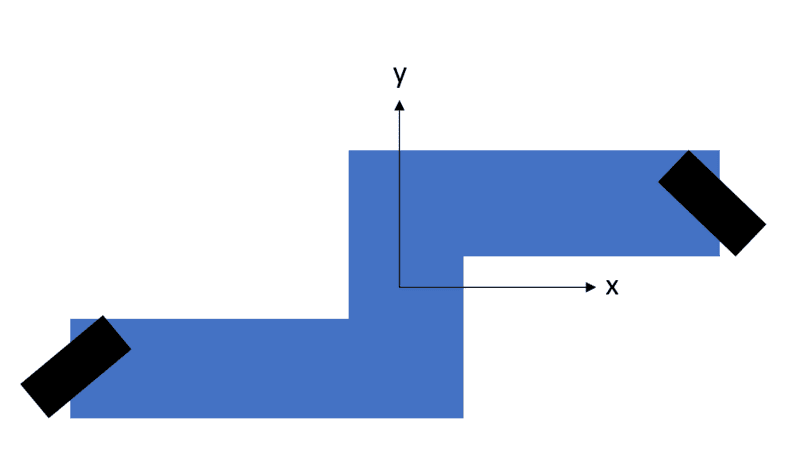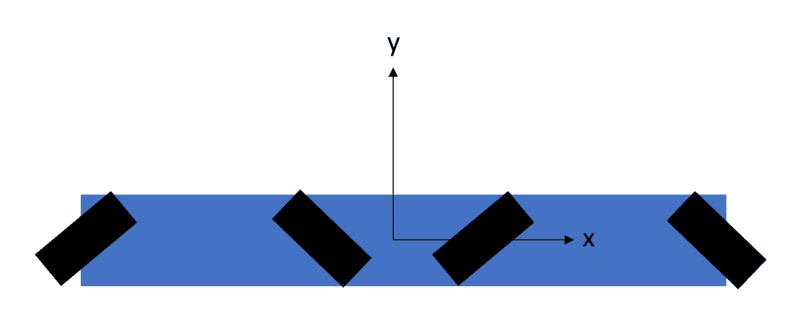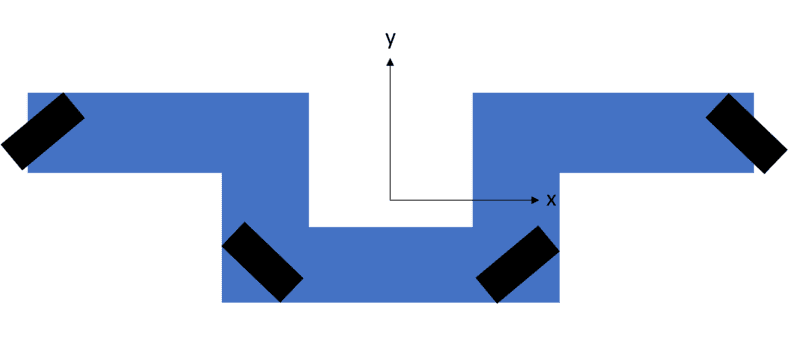# Two Omni Wheel Robot

harpoon
Hello! I have a somewhat complicated robot project that I've simplified here to hopefully get a quick answer. I want to build a robot with two omni-wheels that looks like the following (not drawn to scale), where the black is the omni wheels at 45 degree angles and the blue is the robot body:A couple of questions, assuming the robot (let's call it the first robot) can balance and the body doesn't touch the ground:
1. Can the robot still move in the y- and the x-direction?
2. Assuming the floor is rough and the omni wheel rollers are rubber, will the robot slide if I push the robot body in the y-direction?

Now, for this robot (let's call it the second robot, also not drawn to scale), the arrangement of the omni wheels looks like this:I have the same questions:
1. Can the robot still move in the y- and the x-direction?
2. Assuming the floor is rough and the omni wheel rollers are rubber, will the robot slide if I push the robot body in the y-direction?

In my mind, the robot can still move in the x- and y-directions and won't slide when I push it in the y-direction, but I wasn't 100% sure and wanted to confirm my intuition here. Thank you in advance! Please let me know if I need to clarify anything, and I apologize if this post is confusing.

Welcome to PF.

If the robot has only two wheels, like a Segway, then I don't think it can use Omni Wheels since it has one degree too much directional freedom.

With the motors stopped, it could be pushed to move in a circle about a centre of rotation.

harpoon
Ah, good point, didn't think about that. Thanks! What about something like this?

First robot:Second robot:Same questions:
1. Can the robot still move in the y- and the x-direction?
2. Assuming the floor is rough and the omni wheel rollers are rubber, will the robot slide if I push the robot body in the y-direction?

1. Can the robot still move in the y- and the x-direction?
Do you mean when pushed or when powered?

The Omni wheel has rollers at 90° to the axle, while
the Mecanum wheel has rollers at 45° to the axle.
https://en.wikipedia.org/wiki/Omni_wheel
https://en.wikipedia.org/wiki/Mecanum_wheel

It takes a minimum of three Omni/Mecanum wheels to control directional movement and orientation.

If you want to make an Omni Segway hybrid, your new type 1 could do it.
If you want a stable platform then your new type 2 could do that.
But you should first consider the 3 wheel solutions;
https://en.wikipedia.org/wiki/Kiwi_drive
https://en.wikipedia.org/wiki/Killough_platform

harpoon
Do you mean when pushed or when powered?
Oops, I guess I should have clarified! I meant when powered. Good to know that it works. The nature of my project requires that I use four omni wheels.

Thank you so much for the help!